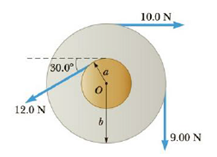Chapter 8, Problem 4P

Chapter
Section
Textbook Problem

Find the net torque on the wheel in Figure P8.4 about the axle through O perpendicular to the page, taking a = 10.0 cm and b = 25.0 cm.Figure P8.4

To determine
The net torque on the wheel.

Explanation
Forces on the wheel are tangential to the various points of contacts on the wheel or alternatively they are perpendicular to the radial vector of the wheel and the torque is about center of the wheel. Assume the torque is positive if it is counter clockwise direction.

Given info: The inner radius of the disk is 10.0cm , outer radius of the disk is 25.0cm , forces are 10.0N , 9.00N , and 12.0N at various points on the wheel.

The formula for the net torque on the wheel is,

τ=aF1b(F2+F3)

• a is inner radius of the wheel.
• b is outer radius of the wheel.
• F1,F2 , and F3 are forces on the wheel.

Substitute 12.0N for F1 , 10.0N for F2 , 9.00N for F3 , 10.0cm for a , and 25

Still sussing out bartleby?

Check out a sample textbook solution.

See a sample solution

The Solution to Your Study Problems

Bartleby provides explanations to thousands of textbook problems written by our experts, many with advanced degrees!

Get Started

Find more solutions based on key concepts